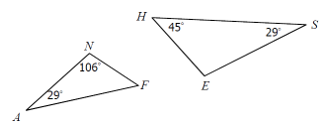Mathematics
Easy

Question

# Determine whether the triangles are similar by AA~, SSS~, SAS~, or not similar.## The correct answer is: AA

### The triangles are similar by AA.Because the triangles have at least two congruent angles.

By angle sum property of a triangle ,we know that sum of interior angles of triangle is 180 degree
So,
In Triangle ANF :Now comparing triangle ANF and EHS
We can easily see they both have all angles same ,so by AA similarity they are similar triangle

### Related Questions to study#### With Turito Foundation.#### Get an Expert Advice From Turito.